4
Thanks

A few words of thanks would be greatly appreciated.

# How to Use Macros in Excel?

Excel is a useful program for creating tables, graphs and making complicated calculations automatically. It might seem complicated at the beginning, but when you get to know Excel’s features, the program becomes very practical and an indispensable tool. One of its most used features is a macro tool that lets you customize and automate frequent actions. In this article, you will learn what macro is and how to use it in Excel.## What Is Macro?

Macro is a handy tool for frequent Excel users that helps to save time and automatically configure some commands. Macro works with VBA (Visual Basic for Application) program codes, but to use them, you don't have to be a programmer at all. All you need to know is just some basic commands and how to use them in practice.

## How to Enable Macros in Excel

To use Macros in Excel, you need to first enable the developer option. To do so, follow the instructions below.

### On Windows

Go to File > Options and choose Customize the Ribbon. Next, in the right column checkmark Developer. Then click OK. Now you will see a Developer tab in your main menu.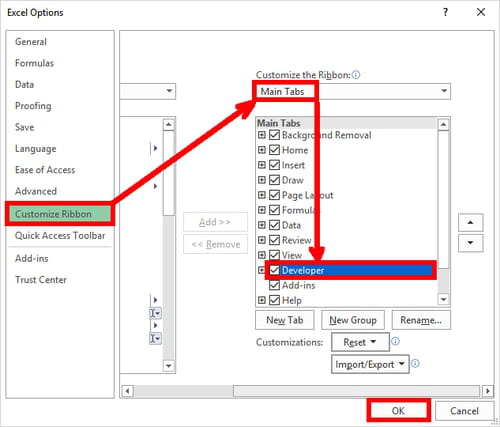### On Mac

Go to Preferences > View and checkmark the Developer tab in Ribbon, Show section.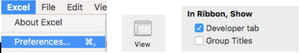## How to Create Macros

Now that you have enabled Macros in your Excel file, you can create your own macros if you use a repetitive task in Excel and want to facilitate it.

To record a macro, go to Developer tab and choose Record Macro.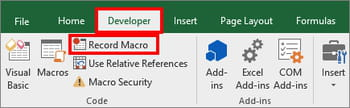In the dialog box fill in the gaps:

In the name section write a name for this macro, choose a shortcut key and the description for the process this macro does. Click OK and perform the action you’d like to save. When you finish, click Stop Recording. Now your macro is saved and you can use it whenever you want.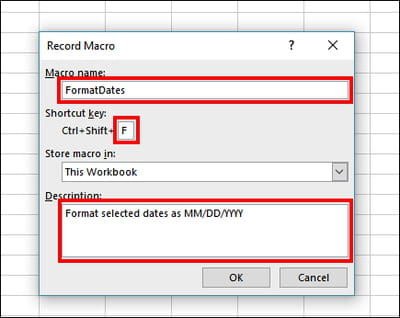## How to Run Macros

To run saved macros, go to Developer tab and select Macros.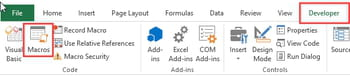In the pop-up window choose the macro you want to use and click Run.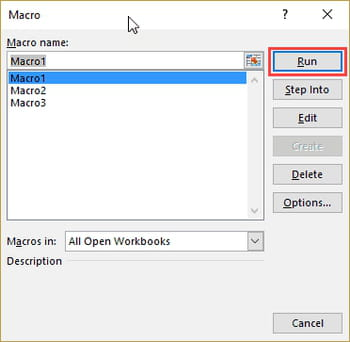## How to Use Specific Macro Codes on Your Excel File

If you want to perform an action that involves a specific macro code, you can use the instructions and the list of the codes below.

### How to Add a Code to the VB

To add a code, open the workbook and press ALT+F11 to open VB Editor or click on Visual Basic in the left corner of the menu. In the Project Explorer choose a workbook to which you wish to add a macro. Right-click on it and choose Insert > Module.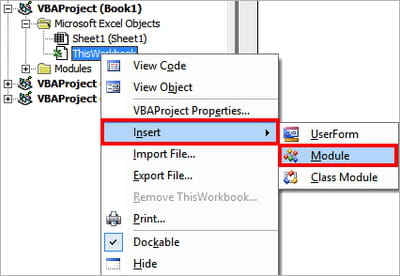Now the new module has to appear in the left tab and you can add a code.Select the module and go to the right tab. Next, paste the code you need. Finally close the window and go back to your workbook. You can find this code in your saved macros. To use this code, follow the instructions on how to run macros outlined above.

### List of Useful Macro Codes For Excel

*To sort the worksheets alphabetically:

Sub SortSheetsTabName()
Application.ScreenUpdating = False
Dim ShCount As Integer, i As Integer, j As Integer
ShCount = Sheets.Count
For i = 1 To ShCount - 1
For j = i + 1 To ShCount
If Sheets(j).Name < Sheets(i).Name Then
Sheets(j).Move before:=Sheets(i)
End If
Next j
Next i
Application.ScreenUpdating = True
End Sub

*To unmerge all the merged cells:

Sub UnmergeAllCells()
ActiveSheet.Cells.UnMerge
End Sub

*To save each worksheet as a separate PDF:

Sub SaveWorkshetAsPDF()
Dim ws As Worksheet
For Each ws In Worksheets
ws.ExportAsFixedFormat xlTypePDF, "C:UsersSumitDesktopTest" & ws.Name & ".pdf"
Next ws
End Sub

*To lock all the cells with formulas:

Sub LockCellsWithFormulas()
With ActiveSheet
.Unprotect
.Cells.Locked = False
.Cells.SpecialCells(xlCellTypeFormulas).Locked = True
.Protect AllowDeletingRows:=True
End With
End Sub

*To automatically insert a row after every row:

Sub InsertAlternateRows()
Dim rng As Range
Dim CountRow As Integer
Dim i As Integer
Set rng = Selection
CountRow = rng.EntireRow.Count
For i = 1 To CountRow
ActiveCell.EntireRow.Insert
ActiveCell.Offset(2, 0).Select
Next i
End Sub

*To highlight the cells that have misspelled words:

Sub HighlightMisspelledCells()
Dim cl As Range
For Each cl In ActiveSheet.UsedRange
If Not Application.CheckSpelling(word:=cl.Text) Then
cl.Interior.Color = vbRed
End If
Next cl
End Sub

*To get only the text part from a string:

Function GetText(CellRef As String)
Dim StringLength As Integer
StringLength = Len(CellRef)
For i = 1 To StringLength
If Not (IsNumeric(Mid(CellRef, i, 1))) Then Result = Result & Mid(CellRef, i, 1)
Next i
GetText = Result
End Function

*To get only the numeric part from a string:

Function GetNumeric(CellRef As String)
Dim StringLength As Integer
StringLength = Len(CellRef)
For i = 1 To StringLength
If IsNumeric(Mid(CellRef, i, 1)) Then Result = Result & Mid(CellRef, i, 1)
Next i
GetNumeric = Result
End Function

Selection.Copy
End Sub

*To add A-Z alphabets in a range:

Dim i As Integer
For i= 65 To 90
ActiveCell.Value= Chr(i)
ActiveCell.Offset(1, 0).Select
Next i
End Sub
Dim i As Integer
For i= 97 To 122
ActiveCell.Value= Chr(i)
ActiveCell.Offset(1, 0).Select
Next i
End Sub

*To delete data in the row while keeping the formula
Sub KeepFormulas() Dim sRow, lCol As Integer sRow = ActiveCell.Row lCol = Cells(sRow, Columns.Count).End(xlToLeft).Column For Each cell In Range(Cells(sRow, 1), Cells(sRow, lCol)) If cell.HasFormula = False Then cell.ClearContents Next cell End Sub

*Delete a string of character in a range of cells

Option Explicit Option Compare Text Sub DeleteWord() Dim Cel As Range, Range As Range Dim Word As String Set Range = Range("B2:B20") '. Word = "Theword" Application.ScreenUpdating = False For Each Cel In Range If Cel Like "*" & Word & "*" Then Cel = Replace(Cel, Word, "") 'To remove the double space that follows .. Cel = Replace(Cel, " ", " ") End If Next Cel Application.ScreenUpdating = True End Sub

*Combine multiple columns into one
Sub test()
Dim j As Long, k As Long, r As Range, dest As Range
j = Range("A1").End(xlToRight).Column
For k = 1 To j
Set r = Range(Cells(1, k), Cells(1, k).End(xlDown))
r.Copy
Set dest = Cells(Rows.Count, "A").End(xlUp).Offset(3, 0)
dest.PasteSpecial
Next k
End Sub

Image © Samuel Sianipar - Unsplash.com

4
Thanks

A few words of thanks would be greatly appreciated.